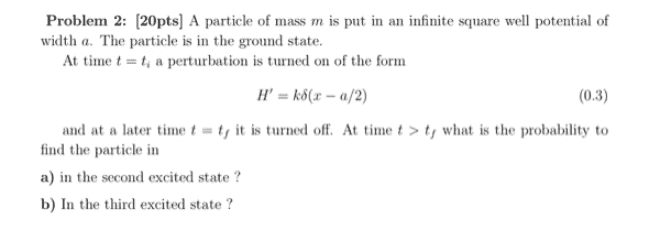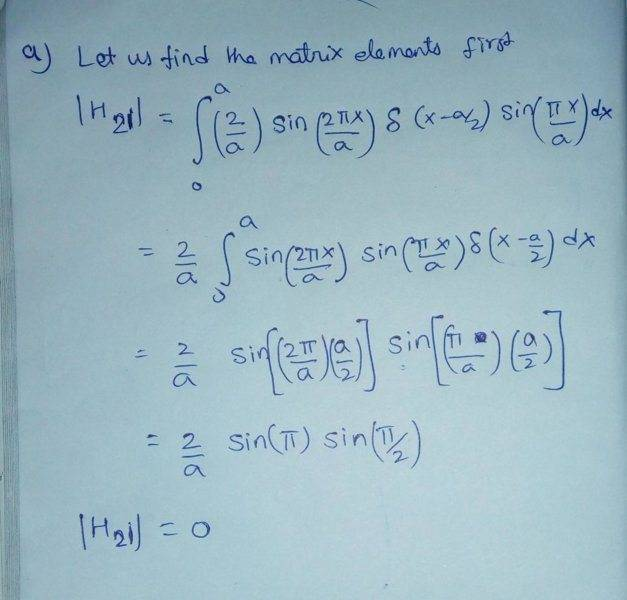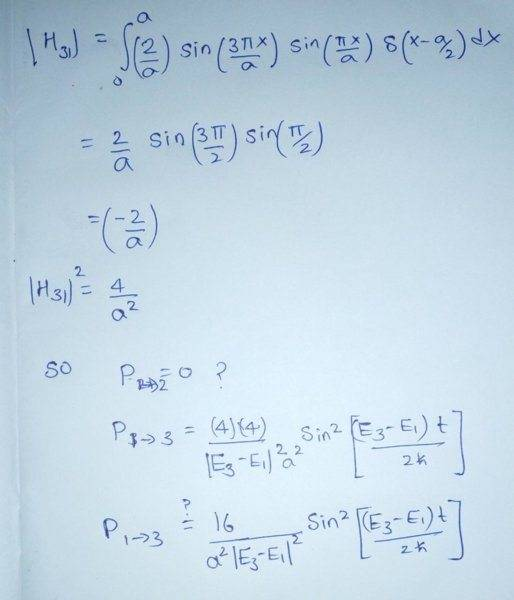# Finding the probability of transition

Muthumanimaran

## Homework Statement

The question is attached below## Homework Equations

$$P_{i \to n}(t)=\frac{|4H_{ni}|^2}{|E_{n}-E_{i}|^2}\sin^2\bigg[ \frac{(E_n-E_i)t}{2\hbar} \bigg]$$

## The Attempt at a Solutionfor simplicity I kept k=1

I don't know whether my approach is correct. That is I am not sure whether I used the correct formula for calculating transition probabilities, I afraid that I have not used the fact the perturbation is turned on at $$t=t_i$$ and turned off at $$t=t_f$$ anywhere in the solution. Any help is appreciated.

#### Attachments

Last edited:

Homework Helper
Gold Member
Your work looks good to me, except you seem to have dropped the factor of ##k## that was part of the perturbation. (Note that your result for ##P_{1 \to 3}## doesn't have the right dimensions for a probability.)

EDIT: Please ignore the parts of my comment related to ##k##. I overlooked your statement: "for simplicity I kept k=1"

To see how ##t_i## and ##t_f## enter the solution, review the meaning of ##t## in
$$P_{i \to n}(t)=\frac{|4H_{ni}|^2}{|E_{n}-E_{i}|^2}\sin^2\bigg[ \frac{(E_n-E_i)t}{2\hbar} \bigg]$$

Last edited:
Muthumanimaran
Your work looks good to me, except you seem to have dropped the factor of ##k## that was part of the perturbation. (Note that your result for ##P_{1 \to 3}## doesn't have the right dimensions for a probability.)

EDIT: Please ignore the parts of my comment related to ##k##. I overlooked your statement: "for simplicity I kept k=1"

To see how ##t_i## and ##t_f## enter the solution, review the meaning of ##t## in
$$P_{i \to n}(t)=\frac{|4H_{ni}|^2}{|E_{n}-E_{i}|^2}\sin^2\bigg[ \frac{(E_n-E_i)t}{2\hbar} \bigg]$$
The time "t" refers to time after "pertubation is turned on"

The relevant expression for probability of transition to find the probability of transition from intial state to final state when the perturbation is turned at t=0, after that perturbation will not be turned off.

But the question asked is to find the transition probability after the perturbation is turned off. Will turning off pertubation after some time will affect the expression for transition amplitude that I mentioned?

Homework Helper
Gold Member
The time "t" refers to time after "pertubation is turned on"

The relevant expression for probability of transition to find the probability of transition from intial state to final state when the perturbation is turned at t=0,
OK. So, how would you alter the expression for the probability so that it corresponds to switching on the perturbation at time ##t = t_i## rather than at ##t = 0##?

Will turning off pertubation after some time will affect the expression for transition amplitude that I mentioned?
No. Your probability expression gives the probability that at some time ##t## (while the perturbation is on) the system would be found in the ##n##th stationary state of the unperturbed system. If the perturbation is switched off at this time, then the system is back to being the unperturbed system. The probability of being in a particular stationary state of the unperturbed system will no longer change with time

•Muthumanimaran
Muthumanimaran
OK. So, how would you alter the expression for the probability so that it corresponds to switching on the perturbation at time ##t = t_i## rather than at ##t = 0##?

No. Your probability expression gives the probability that at some time ##t## (while the perturbation is on) the system would be found in the ##n##th stationary state of the unperturbed system. If the perturbation is switched off at this time, then the system is back to being the unperturbed system. The probability of being in a particular stationary state of the unperturbed system will no longer change with time
Thanks now I understand what you are trying to say.